# Review: BoWNet, Bags of Visual Words Predictions

## Teacher-Student-Based Self-Supervised Learning Using Bag of Visual Words, Outperforms MoCo, PIRL, & Jigsaw

Learning Representations by Predicting Bags of Visual Words
BoWNet
, by Valeo.ai, and University of Crete
2020 CVPR, Over 40 Citations (Sik-Ho Tsang @ Medium)
Self-Supervised Learning, Unsupervised Learning, Teacher Student, Representation Learning, Image Classification, Object Detection

• A self-supervised approach is proposed based on spatially dense image descriptions that encode discrete visual concepts, called visual words.
• The feature maps of a first pretrained self-supervised convnet is quantized, over a k-means based vocabulary.
• Then, another convnet is trained to predict the histogram of visual words of an image (i.e., its Bag-of-Words representation) given as input a perturbed version of that image.
• Thus, BoWNet forces the convnet to learn perturbation-invariant and context-aware image features.

# Outline

1. BoWNet
2. Experimental Results

# 1. BoWNet

• The goal is to learn in an unsupervised way a feature extractor or convnet model Φ(·) parameterized by θ that, given an image x, produces a “good” image representation Φ(x) for other downstream tasks.

## 1.1. Building Spatially Dense Discrete Descriptions q(x)

• Given a training image x, the first step for our method is to create a spatially dense visual words-based description q(x) using the pre-trained convnet ˆΦ(·).
• Self-supervised ImageNet-pretrained RotNet is used as ˆΦ(·).
• Specifically, let ˆΦ(x) be a feature map (with ˆc channels and ˆh×ˆw spatial size) produced by ˆΦ(·) for input x, and ˆu(x) the ˆc-dimensional feature vector at the location u ∈ {1, · · · ,U}, where Uh·ˆw.
• To generate the description q(x) = [q1(x), …, qU(x)], ˆΦ(x) is densely quantized using a predefined vocabulary V = [v1, …, vK] of ˆc-dimensional visual word embeddings, where K is the vocabulary size.

For each position u, the corresponding feature vector ˆΦu(x) is assigned to its closest (in terms of squared Euclidean distance) visual word embedding qu(x):

• where the vocabulary V is learned by applying the k-means algorithm with K clusters to a set of feature maps extracted from the dataset X, i.e., by optimizing the following objective:
• where the visual word embedding vk is the centroid of the k-th cluster.

## 1.2. Generating Bag-of-Words Representations y(x)

• There are two ways: Histogram version and Binary version.
• Histogram version: A K-dimensional vector whose k-th element yk(x) either encodes the number of times the k-th visual word appears in image x:
• Binary version: or indicates if the k-th visual word appears in image x:
• where 1[·] is the indicator operator.
• The binary version is used for ImageNet and the histogram version for CIFAR-100 and MiniImageNet.
• To convert y(x) into a probability distribution over visual words, y(x) is L1-normalized.

Thus, the resulting y(x) can thus be perceived as a soft categorical label of x for the K visual words.

## 1.3. Learning to “Reconstruct” BoW Ω(Φ(~x))

Given an image x, a perturbation operator g(·) is applied onto i, to get the perturbed image ˜x=g(x).

Then, the model is trained to predict/“reconstruct” the BoW epresentation y(x) of the original unperturbed image x from ˜x.

• Specifically, for g(.), it consists of color jittering, random grayscale, random crop, scale distortion, and horizontal flipping.
• In addition, CutMix augmentation is also used.

Thus, the convnet must learn image features that (1) are robust w.r.t. the applied perturbations and at the same time (2) allow predicting the visual words of the original image, even for image regions that are not visible to the convnet due to cropping.

• The feature representation produced by model Φ(·) is c-dimensional.
• Ω(.) takes this feature as input and outputs a K-dimensional softmax distribution over the K visual words of the BoW representation. This prediction layer is implemented with a linear-plus-softmax layer:
• W=[w1, …, wK] are the K c-dimensional weight vectors (one per visual word) of the linear layer. But instead using W directly, a L2-normalized version of W is used:
• with a unique learnable magnitude γ for all the weight vectors.
• The reason for this reparametrization of the linear layer is because the distribution of visual words in the dataset tends to be unbalanced and, so, without such a reparametrization the network would attempt to make the magnitude of each weight vector proportional to the frequency of its corresponding visual word.

## 1.4. Self-Supervised Training Objective

• The training loss that is to minimize for learning the convnet model Φ(·) is the expected cross-entropy loss between the predicted softmax distribution Ω(Φ(˜x)) and the BoW distribution y(x):
• The self-supervised method can be applied iteratively, using each time the previously trained model ˆΦ(·) for creating the BoW representation.
• The model learned from the first iteration already achieves very strong results. As a result, only a few more iterations (e.g., one or two) might be applied after that.

# 2. Experimental Results

## 2.1. CIFAR-100 & MiniimageNet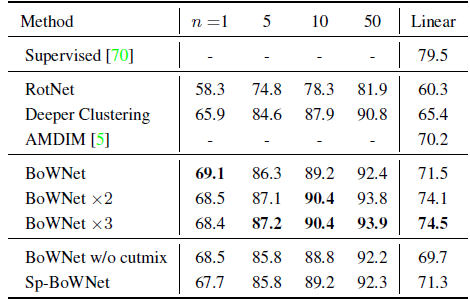CIFAR-100 linear classifier and few-shot results with WRN-28–10.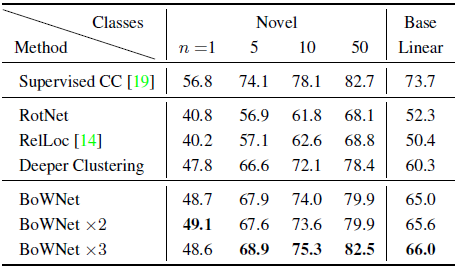MiniImageNet linear classifier and few-shot results with WRN-28–4.
• K=2048 visual words is used.

By comparing BoWNet with RotNet, BoWNet improves all the evaluation metrics by at least 10 percentage points, which is a very large performance improvement.

• Applying BoWNet iteratively (entries BoWNet ×2 and BoWNet ×3) further improves the results (except the 1-shot accuracy).
• Also, BoWNet outperforms by a large margin the CIFAR-100 linear classification accuracy of the recent AMDIM .
• Comparing with Deeper Clustering, it got several absolute percentage points lower linear classification accuracy, which illustrates the advantage of using BoW as targets for self-supervision instead of the single cluster id of an image.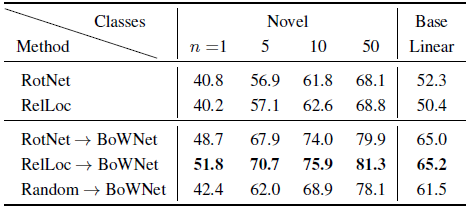MiniImageNet linear classifier and few-shot results with WRN-28–4. Impact of base convnet.

RelLoc→BoWNet achieves equally strong or better results than in the RotNet→BoWNet case.

• It is noted that with a random base convnet, the performance of BoWNet drops. However, BoWNet still is significantly better than RotNet and RelLoc.

## 2.2. PASCAL VOC 2007 Classification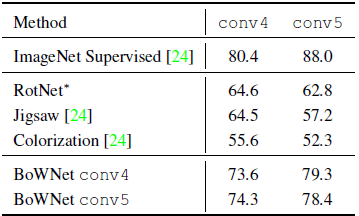VOC07 image classification results for ResNet-50 Linear SVMs.
• K=20000 visual words is used.

BoWNet outperforms all prior methods.

• Interestingly, conv4-based BoW leads to better classification results for the conv5 layer of BoWNet, and conv5-based BoW leads to better classification results for the conv4 layer of BoWNet.

## 2.3. ImageNet and Place 205 Classification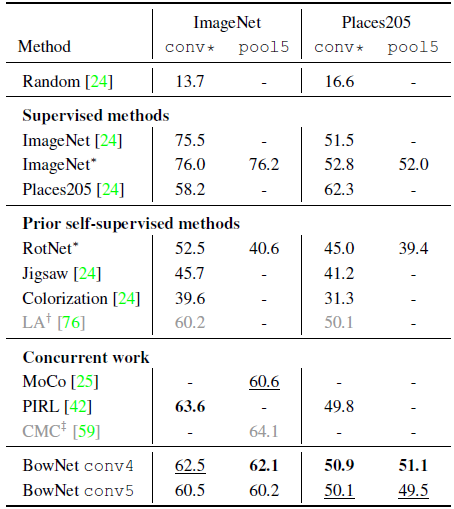ResNet-50 top-1 center-crop linear classification accuracy on ImageNet and Places205.

BoWNet outperforms all prior self-supervised methods by significant margin.

• Furthermore, the accuracy gap on Places205 between our ImageNet-trained BoWNet representations and the ImageNet-trained supervised representations is only 0.9 points in pool5. This demonstrates that the self-supervised representations have almost the same generalization ability to the “unseen” (during training) Places205 classes as the supervised ones.
• Concurrent MoCo  and PIRL  methods are also compared.

BoWNet outperforms MoCo on ImageNet.

When compared to PIRL, BoWNet has around 1 point higher Places205 accuracy but 1 point lower ImageNet accuracy.

## 2.4. PASCAL VOC 2007 Object Detection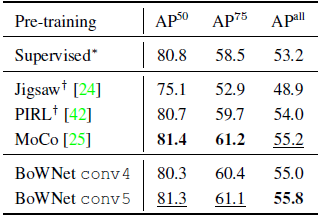Object detection with Faster R-CNN fine-tuned on VOC trainval07+12.
• Faster R-CNN with a ResNet-50 backbone is used. The pre-trained BoWNet is fine-tuned on trainval07+12 and evaluated on test07.

Both BoWNet variants exhibit strong performance.

• BoWNet outperforms the supervised ImageNet pretrained model, which is fine-tuned in the same conditions as BoWNet. So, the self-supervised representations generalize better to the VOC detection task than the supervised ones.

The BoWNet framework exhibits a Teacher-Student architecture where contrastive learning is not required.

## Reference

[2020 CVPR] [BoWNet]
Learning Representations by Predicting Bags of Visual Words

## Unsupervised/Self-Supervised Learning

1993–2017 … 2018 [RotNet/Image Rotations] [DeepCluster] [CPC/CPCv1] [Instance Discrimination] 2019 [Ye CVPR’19] 2020 [CMC] [MoCo] [CPCv2] [PIRL] [SimCLR] [MoCo v2] [iGPT] [BoWNet]

--

--